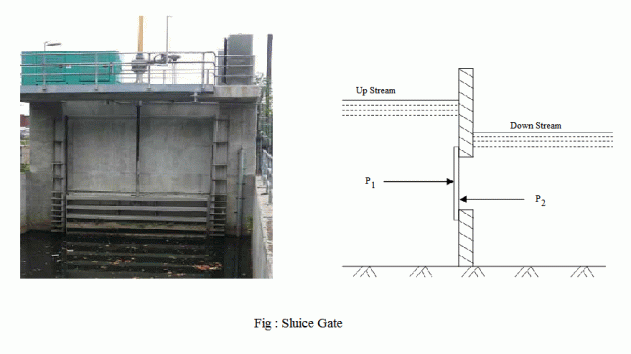I have forgotten

•http://facebook.com/
•https://www.google.com/accounts/o8/id
•https://me.yahoo.com

# Sluice Gates

Water Pressure on Sluice GatesView version details

### Key Facts

Gyroscopic Couple: The rate of change of angular momentum () =(In the limit).
•= Moment of Inertia.
•= Angular velocity
•= Angular velocity of precession.

Blaise Pascal (1623-1662) was a French mathematician, physicist, inventor, writer and Catholic philosopher.

Leonhard Euler (1707-1783) was a pioneering Swiss mathematician and physicist.

## Introduction

A sluice gate is provided, in the path of a river or a stream, to regulate the flow of water. For doing so, the sluice gate is made to move up and down with the help of rollers fixed to the vertical plates (called skin plates) which travel on vertical rails called guides. These rails are fixed on piers or vertical walls as shown in Figure. In between these two skin plates , a number of I-beams are provided horizontally to withstand the water pressure. As the water pressure varies with the depth, the spacing between the I-beams is lesser at the bottom than that at the top of the sluice gate.Water acts on both sides of a sluice gate as shown in figure. The resultant pressure on the sluice gate is obtained as follows:

Let,
•= Wetted area on the upstream of the gate
•= Depth of center of gravity of the wetted area on the upstream side of the gate, and
•= Corresponding values for the downstream side of the gatePressure on the upstream side of the gate,Similarly, pressure on the downstream side of the gate,As the two pressure are acting in opposite directions, therefore the resultant pressure,The point of application of the resultant pressure (P) may be found out by taking moments of the two pressure (P1 and P2) about the top or bottom of the gate.

Example:
[metric]
##### Example - Water Pressure on Sluic Gate
Problem
A vertical sluice gate 3m wide and 2.5m deep contains water on both of its sides. On the upstream side, the water is 5m deep and on the downstream side it is 2m deep from the bottom of the sluice. What is the resultant pressure on the gate?

Workings
Given,
• Width of the sluice gate, b = 3m
• Depth of the sluice gate = 2.5m
• Depth of water on the upstream side = 5m
• Depth of water on the downstream side = 2m

The area of sluice gate submerged on the upstream side,

and depth of c.g. of the sluice gate from the water level on the upstream side,

Similarly, area of sluice gate submerged on the downstream side,

and depth of c.g. of the sluice gate from the water level on the downstream side,

Pressure due to water on the upstream side of the gate,

and Pressure due to water on the downstream side of the gate,

Resultant pressure on the gate,
Solution
Resultant pressure on the gate = 217 KN